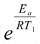# Another LaTeX formatting question

• LaTeX
Mentor
So I was answering in a thread here and what I got was ugly:

$$k_1 = e^{\frac {E_a} {RT_1}}$$

What I don't like is that the exponential part is so small it is barely readable (checked in Opera, Firefox, IE and Chrome). I can write it as

$$k_1 = exp\left({\frac {E_a} {RT_1}}\right)$$

and it definitely looks better, but sometimes I feel like writing ex instead of exp(x) makes the equation easier to follow - at least for me it is easier to see and group exponents when they are all in superscript position.

Is there a way of making the exponential part larger?

For example OpenOffice formats it asStill not perfect, but closer to what I am thinking about.

Any ideas?

#### Attachments

Gold Member
$$\large k_1 = e^{\frac {E_a} {RT_1}}$$

$$\LARGE k_1 = e^{\frac {E_a} {RT_1}}$$

Increasing the size helps sometimes although it probably isn't too practical.

EDIT:

This here doesn't look too bad $$k_1 = e^{\LARGE\frac {E_a} {RT_1}}$$

Staff Emeritus
$$x^{x}$$
Note how the superscript x is in a smaller font, that dictated by \scriptstyle.

$$a^{a^a}$$
Now note how the font sizes get progressively smaller. The font size goes from that dictated by \displaystyle to \scriptstyle to \scriptscriptsyle (which is where things stop).

$$e^{\frac{E_a}{RT_1}}$$
That "getting progressively smaller" is what is happening here. Both the superscript and \frac act to reduce the font by one level. The end result is that Ea and RT1 are in that tiny \scriptscriptsyle font.

One way to overcome this is to force the numerator and denominator to be in the size dictated by \scriptstyle:
$$e^{\frac{E_a}{RT_1}}$$

Alternatively, just don't use the fractional form:
$$e^{E_a/(RT_1)}$$

With some abuse of notation,
$$e^{E_a/RT_1}$$

Mentor
ThanksNo idea which one I will use, but it is nice to have some options to select from.